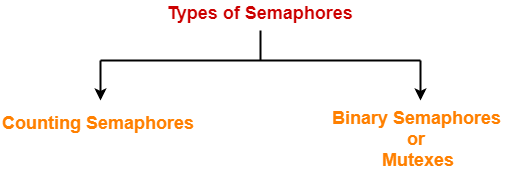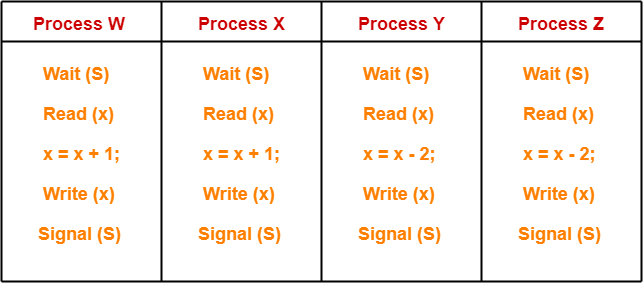# Semaphore in OS | Practice Problems

## Semaphore in OS-

Before you go through this article, make sure that you have gone through the previous article on Semaphores in OS.

We have discussed-

• A semaphore is a simple integer variable used to provide synchronization among the processes.
• There are mainly two types of semaphores-In this article, we will discuss practice problems based on counting semaphores.

## Problem-01:

A counting semaphore S is initialized to 10. Then, 6 P operations and 4 V operations are performed on S. What is the final value of S?

## Solution-

We know-

• P operation also called as wait operation decrements the value of semaphore variable by 1.
• V operation also called as signal operation increments the value of semaphore variable by 1.

Thus,

Final value of semaphore variable S

= 10 – (6 x 1) + (4 x 1)

= 10 – 6 + 4

= 8

## Problem-02:

A counting semaphore S is initialized to 7. Then, 20 P operations and 15 V operations are performed on S. What is the final value of S?

## Solution-

We know-

• P operation also called as wait operation decrements the value of semaphore variable by 1.
• V operation also called as signal operation increments the value of semaphore variable by 1.

Thus,

Final value of semaphore variable S

= 7 – (20 x 1) + (15 x 1)

= 7 – 20 + 15

= 2

## Problem-03:

A shared variable x, initialized to zero, is operated on by four concurrent processes W, X, Y, Z as follows. Each of the processes W and X reads x from memory, increments by one, stores it to memory and then terminates. Each of the processes Y and Z reads x from memory, decrements by two, stores it to memory, and then terminates. Each process before reading x invokes the P operation (i.e. wait) on a counting semaphore S and invokes the V operation (i.e. signal) on the semaphore S after storing x to memory. Semaphore S is initialized to two. What is the maximum possible value of x after all processes complete execution?

1. -2
2. -1
3. 1
4. 2

## Solution-

The given question may be pictorially represented as-Initially, counting semaphore S is initialized with value 2.

Now, We have been asked the maximum possible value of x after all the processes complete execution.

Clearly,

• Processes W and X increments the value of x.
• Processes Y and Z decrements the value of x.

To obtain the maximum value of x, the processes must execute in such a way that-

• Only the impact of the processes W and X remains on the value of x.
• The impact of processes Y and Z gets lost on the value of x.

### STRATEGY

• First of all, make the process W read the value of x = 0.
• Then, preempt the process W.
• Now, schedule process Y and process Z to execute one by one.
• After executing them, reschedule process W.
• Now, when process W gets scheduled again, it starts with value x = 0 and increments this value.
• This is because before preemption it had read the value x = 0.
• The updates from the processes Y and Z gets lost.
• Later, execute process X which again increments the value of x.
• Here, the lost update problem has been exploited.

This is achieved as-

### Step-01:

• Process W arrives.
• It executes the wait(S) operation and the value of S decrements by 1. Now, S = 1.
• It reads the value x = 0.
• It gets preempted.

### Step-02:

• Process Y arrives.
• It executes the wait(S) operation and the value of S decrements by 1. Now, S = 0.
• It reads the value x = 0.
• It decrements the value of x by 2. Now, x = 0 – 2 = -2.
• It writes the value x = -2 in the memory.
• It executes the signal(S) operation and the value of S increments by 1. Now, S = 1.
• Now, execution of process Y is completed.

### Step-03:

• Process Z arrives.
• It executes the wait(S) operation and the value of S decrements by 1. Now, S = 0.
• It reads the value x = -2.
• It decrements the value of x by 2. Now, x = – 2 – 2 = -4.
• It writes the value x = -4 in the memory.
• It executes the signal(S) operation and the value of S increments by 1. Now, S = 1.
• Now, execution of process Z is completed.

### Step-04:

• Process W gets scheduled again.
• It resumes its execution from where it left.
• Now, it increments the value of x by 1. Now, x = 0 + 1 = 1.
• It writes the value x = 1 in the memory.
• It executes the signal(S) operation and the value of S increments by 1. Now, S = 2.
• Now, execution of process W is completed.

### Step-05:

• Process X arrives.
• It executes the wait(S) operation and the value of S decrements by 1. Now, S = 1.
• It reads the value x = 1.
• It increments the value of x by 1. Now, x = 1 + 1 = 2.
• It writes the value x = 2 in the memory.
• It executes the signal(S) operation and the value of S increments by 1. Now, S = 2.
• Now, execution of process X is completed.

Thus,

• Final value of x = 2.
• This is the maximum possible value of x that can be achieved after executing all the 4 processes.
• Option (D) is correct.

## Problem-04:

In problem-03, what is the minimum possible value of x after all processes complete execution?

1. -4
2. -2
3. 2
4. 4

## Solution-

To obtain the minimum value of x, the processes must execute in such a way that-

• Only the impact of the processes Y and Z remains on the value of x.
• The impact of processes W and X gets lost on the value of x.

This can be achieved if processes execute in the following manner-

### Step-01:

• Process Y arrives.
• It executes the wait(S) operation and the value of S decrements by 1. Now, S = 1.
• It reads the value x = 0.
• It gets preempted.

### Step-02:

• Process W arrives.
• It executes the wait(S) operation and the value of S decrements by 1. Now, S = 0.
• It reads the value x = 0.
• It increments the value of x by 1. Now, x = 0 + 1 = 1.
• It writes the value x = 1 in the memory.
• It executes the signal(S) operation and the value of S increments by 1. Now, S = 1.
• Now, execution of process W is completed.

### Step-03:

• Process X arrives.
• It executes the wait(S) operation and the value of S decrements by 1. Now, S = 0.
• It reads the value x = 1.
• It increments the value of x by 1. Now, x = 1 + 1 = 2.
• It writes the value x = 2 in the memory.
• It executes the signal(S) operation and the value of S increments by 1. Now, S = 1.
• Now, execution of process X is completed.

### Step-04:

• Process Y gets scheduled again.
• It resumes its execution from where it left.
• Now, it decrements the value of x by 2. Now, x = 0 – 2 = -2.
• It writes the value x = -2 in the memory.
• It executes the signal(S) operation and the value of S increments by 1. Now, S = 2.
• Now, execution of process Y is completed.

### Step-05:

• Process Z arrives.
• It executes the wait(S) operation and the value of S decrements by 1. Now, S = 1.
• It reads the value x = -2.
• It decrements the value of x by 2. Now, x = -2 – 2 = -4.
• It writes the value x = -4 in the memory.
• It executes the signal(S) operation and the value of S increments by 1. Now, S = 2.
• Now, execution of process Z is completed.

Thus,

• Final value of x = -4.
• This is the minimum possible value of x that can be achieved after executing all the 4 processes.

Thus, Option (A) is correct.

To watch video solutions and practice other problems,

Watch this Video Lecture

Next Article- Binary Semaphores

Get more notes and other study material of Operating System.

Watch video lectures by visiting our YouTube channel LearnVidFun.

SummaryArticle Name
Semaphore in OS | Practice Problems
Description
Practice Problems based on Semaphore in OS. In operating system, there are two types of semaphores- Counting Semaphore & Binary Semaphore also called as mutex.
Author
Publisher Name
Gate Vidyalay
Publisher Logo